# PostgreSQL FOREIGN KEY constraint

## FOREIGN KEY constraint

A FOREIGN KEY constraint contains the value in a column or combination of columns which must be appearing in the same column or group of columns in another table.

The PostgreSQL FOREIGN KEY is a combination of columns with values based on the primary key values from another table. A foreign key constraint, also known as Referential integrity Constraint, specifies that the values of the foreign key correspond to actual values of the primary key in the other table.

## PostgreSQL FOREIGN KEY example

Let us consider two tables vendors and items to illustrate the FOREIGN KEY in PostgreSQL. The vendors table contain a primary key vendor_code and the items table contain the primary key item_code.

## SQL

``````CREATE TABLE vendors(vendor_code integer PRIMARY KEY,vendor_name character(35),vendor_city character(15),vendor_country character(20));
```
```

## The table structure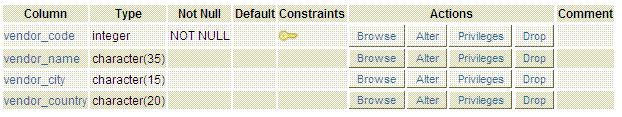## SQL

``````CREATE TABLE items(item_code integer,item_name character(35),purchase_unit character(10),sale_unit character(10),purchase_price numeric(10,2),sale_price numeric(10,2),PRIMARY KEY (item_code,item_name));
```
```

## The table structure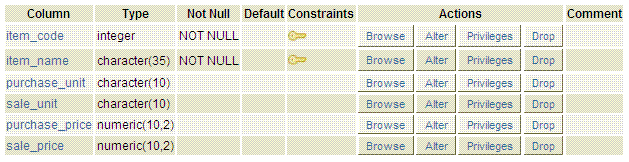Let us assume that we want to take order with a unique order_no and those items in orders table which must be appearing in items table, the following SQL can be executed.

## SQL

``````CREATE TABLE orders(ord_no integer PRIMARY KEY,ord_date date,item_code integer REFERENCES items(item_code),item_grade character(1),ord_qty numeric,ord_amount numeric);
```
```

## Pictorial representation of FOREIGN KEY constraint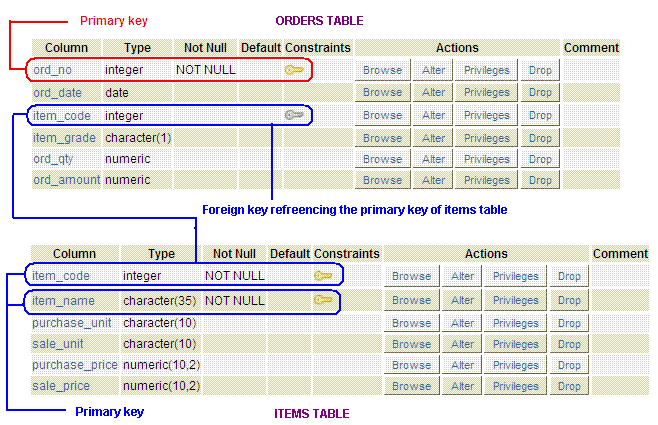## Explanation

The above example and picture explain that the item_code column of orders table can only contain the values which appearing in the item_code column of items table.

Here orders table is the referencing table and items is the referenced table and the item_code or orders table is the referencing column and the item_code of items table is the referenced column.

OR the above can be written as bellow -

``````CREATE TABLE orders(ord_no integer PRIMARY KEY,ord_date date,item_code integer REFERENCES items,item_grade character(1),ord_qty numeric,ord_amount numeric);
```
```

because if mention no column(s) as reference the primary key(s) of referenced table is used as referenced column(s).

## PostgreSQL FOREIGN KEY constraint on group of columns

Let us assume that, we want to take order with a unique order_no and those items with their code in orders table which must be appearing in items table, the following SQL can be executed.

## SQL

``````CREATE TABLE orders(ord_no integer PRIMARY KEY,ord_date date,item_code integer ,item_name character(35),item_grade character(1),ord_qty numeric,ord_amount numeric,FOREIGN KEY (item_code,item_name) REFERENCES items(item_code,item_name));
```
```

## Pictorial representation of FOREIGN KEY constraint on group of columns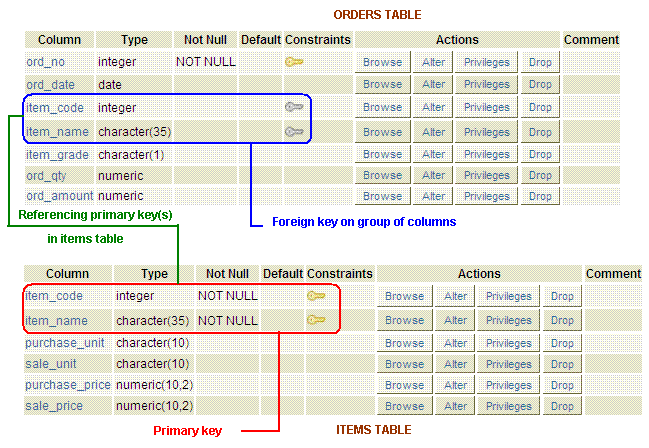## Explain

The above example shows the orders table created with two constraints one PRIMARY KEY and one FOREIGN KEY. The PRIMARY KEY constraint contain one column and the FOREIGN KEY constrain contain a group of columns.

The point to be remembered that, type and number of columns in FOREIGN KEY must be matched with the type and number of columns with the referenced columns in referenced table.

## Table contain more than one PostgreSQL FOREIGN KEY constraint

Assume that the structure of items table is -

``````CREATE TABLE items(item_code integer PRIMARY KEY,item_name character(35),purchase_unit character(10),sale_unit character(10),purchase_price numeric(10,2),sale_price numeric(10,2));
```
```

Let us assume that, we want to take order those items and vendor in orders table thus that the items must be appearing in items table and vendors must appearing in the vendors table, the following SQL can be executed.

## SQL

``````CREATE TABLE orders(ord_no integer,ord_date date,item_code integer REFERENCES items,vendor_code integer REFERENCES vendors,item_grade character(1),ord_qty numeric,ord_amount numeric,PRIMARY KEY (item_code,vendor_code));
```
```

## Pictorial representation of more than one PostgreSQL FOREIGN KEY constraint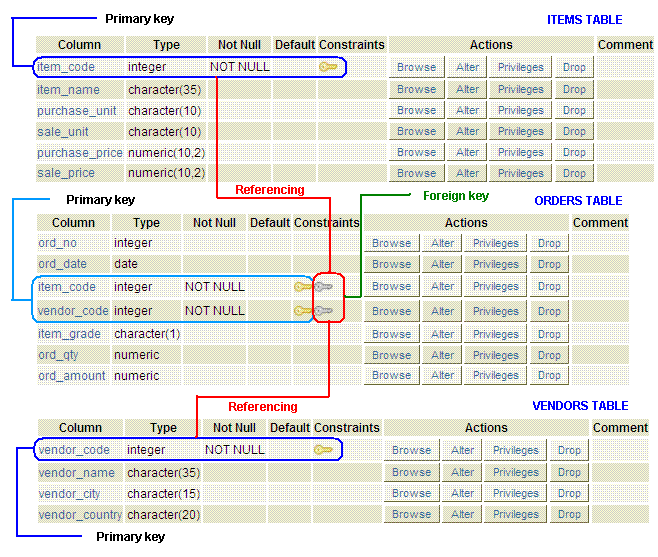## Explain

The above example shows the orders table contain FOREIGN KEY constraint which referencing form two tables. One is referencing from items table that means only those item_code will contain in orders table which is appearing in items table and the second one is referencing the vendors table, that means only those vendor_code will contain in orders table which is appearing in vendors table. A primary key also is creating in orders table which contains a group of columns.

﻿